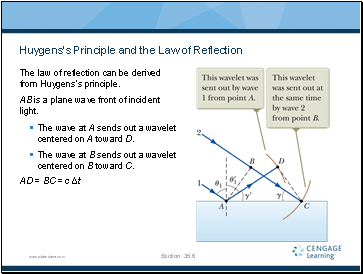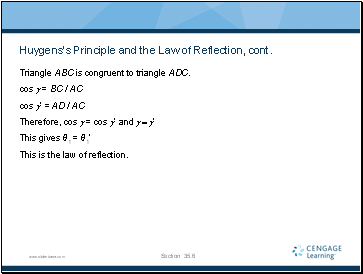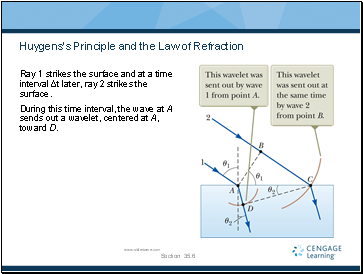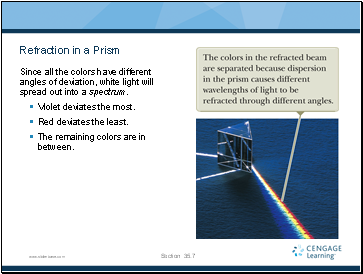# The Nature of Light and the Laws of Geometric OpticsPage 7

#### WATCH ALL SLIDES

Section 35.6

Slide 42## Huygens’s Principle and the Law of Reflection

The law of reflection can be derived from Huygens’s principle.

AB is a plane wave front of incident light.

The wave at A sends out a wavelet centered on A toward D.

The wave at B sends out a wavelet centered on B toward C.

AD = BC = c Δt

Section 35.6

Slide 43## Huygens’s Principle and the Law of Reflection, cont.

Triangle ABC is congruent to triangle ADC.

cos g = BC / AC

cos g’ = AD / AC

Therefore, cos g = cos g’ and g = g’

This gives θ1 = θ1’

This is the law of reflection.

Section 35.6

Slide 44## Huygens’s Principle and the Law of Refraction

Ray 1 strikes the surface and at a time interval Δt later, ray 2 strikes the surface.

During this time interval, the wave at A sends out a wavelet, centered at A, toward D.

Section 35.6

Slide 45## Huygens’s Principle and the Law of Refraction, cont.

The wave at B sends out a wavelet, centered at B, toward C.

The two wavelets travel in different media, therefore their radii are different.

From triangles ABC and ADC, we find

Section 35.6

Slide 46## Huygens’s Principle and the Law of Refraction, final

The preceding equation can be simplified to

This is Snell’s law of refraction.

Section 35.6

Slide 47## Dispersion

For a given material, the index of refraction varies with the wavelength of the light passing through the material.

This dependence of n on λ is called dispersion.

Snell’s law indicates light of different wavelengths is bent at different angles when incident on a refracting material.

Section 35.7

Slide 48## Variation of Index of Refraction with Wavelength

The index of refraction for a material generally decreases with increasing wavelength.

Violet light bends more than red light when passing into a refracting material.

Section 35.7

Slide 49## Refraction in a Prism

Since all the colors have different angles of deviation, white light will spread out into a spectrum.

Violet deviates the most.

Red deviates the least.

The remaining colors are in between.

Section 35.7

Slide 50

Go to page:
1  2  3  4  5  6  7  8  9